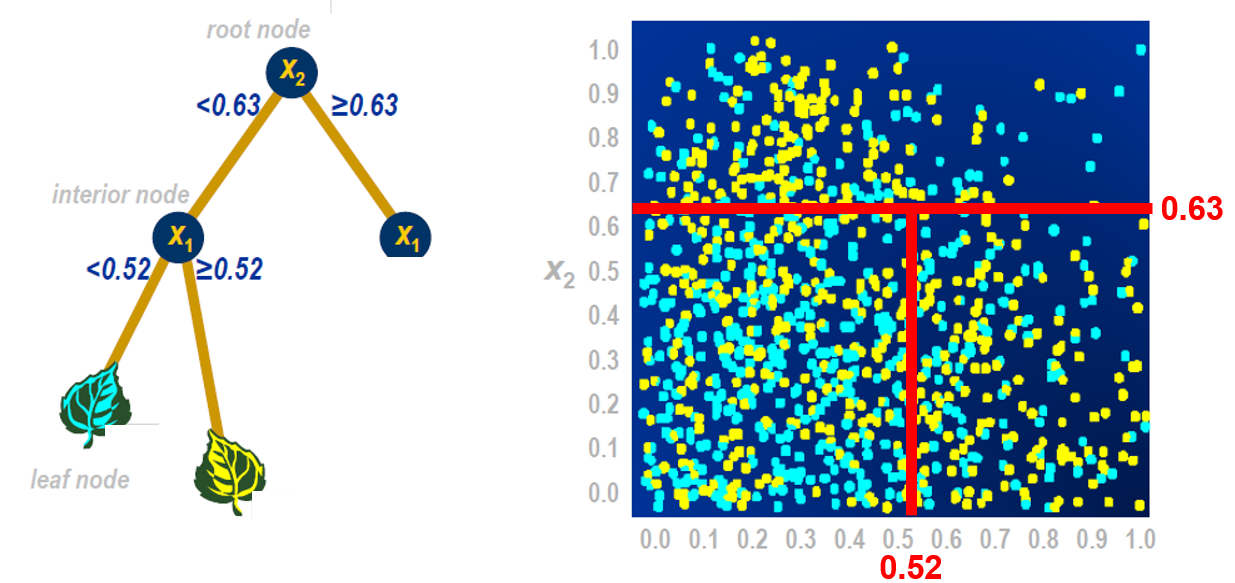## Tree-related Models: Supervised Learning in SAS Visual Data Mining and Machine Learning

Started ‎03-22-2017 by
Modified ‎06-14-2017 by
Views 2,145

In a previous post, I summarized the supervised learning models (the neural networks). In this post, I'll explore tree-related models.

Decision Trees (PROC TREESPLIT)

PROC TREESPLIT procedure builds decision trees in SAS Viya. The tree may be either:

• a classification tree, which models a categorical target, or
• a regression tree, which models a continuous target.

The model is expressed as a series of if-then statements. For each tree, you specify a target (dependent, response) variable and one or more input (independent, predictor) variables. The input variables for tree models can be categorical or continuous. The initial node is called the root node, and the terminal nodes are called leaves. Partitioning is done repeatedly, starting with the root node, which contains all the data, and continuing to split the data until a stopping criterion is met. At each step, the parent node is split into two or more child nodes by selecting an input variable and a split value for that variable.Various measures, such as the Gini index, entropy, and residual sum of squares, can be used to assess candidate splits for each node. The process of building a decision tree begins with growing a large, full tree. The full tree can overfit the training data, resulting in a model that does not adequately generalize to new data. To prevent overfitting, the full tree is often pruned back to a smaller subtree that balances the goals of fitting training data and predicting new data. Two commonly applied approaches for finding the best subtree are cost-complexity pruning  and C4.5 pruning.

Compared with other regression and classification methods, decision trees have the advantage of being easy to interpret and visualize, especially when the tree is small. Tree-based methods scale well to large data, and they offer various methods of handling missing values, including surrogate splits.

However, decision trees do have limitations. Regression trees fit response surfaces that are constant over rectangular regions of the predictor space, so they often lack the flexibility needed to capture smooth relationships between the predictor variables and the response. Another limitation of tree models is that small changes in the data can lead to very different splits, and this undermines the interpretability of the model. Random forest models address some of these limitations.

Random Forest Models (PROC FOREST)A random forest is just what the name implies. It’s a bunch of decision trees – each with a randomly selected subset of the data – all combined into one result. Using a random forest helps address the problem of overfitting inherent to an individual decision tree.

SAS PROC FOREST creates a random forest using literally hundreds of decision trees. Each decision tree uses a different set of data, which is subset from the original data as follows:

1. Subset the rows (observations) to build training data set
2. Subset the features (variables) available to each splitting node

SAS PROC FOREST uses sampling with replacement per Leo Breiman’s bagging algorithm (Breiman 1996, 2001). You may hear the term “ensemble model” for random forest models and gradient boosting models; these are techniques that combine other models.Gradient boosting creates an ensemble model of weak prediction models (in this case, decision trees) in a stage-wise, iterative, sequential manner. Gradient boosting algorithms convert weak learners to strong learners. One advantage of gradient boosting is that it can reduce bias and variance in supervised learning.

All points begin with the same weight. Points classified correctly are given a lower weight and those classified incorrectly are given a higher weight. Now the model focuses on high weight points and classifies them correctly. However, others that were classified correctly in the first iteration are now misclassified. This process continues for many iterations. In the end, all models are given a weight depending on their accuracy, and the model results are combined into one consolidated result.

Hyperparameter Tuning

Hyperparameter tuning is available in the three tree-based procedures, TREESPLIT, FOREST, and GRADBOOST, to find the best values for various options.  These include the splitting criterion, maximum depth, and number of bins in PROC TREESPLIT; the fraction of training data to sample, maximum tree depth, number of trees, and number of variables to consider for each split in PROC FOREST; and the L1 and L2 regularization parameters, learning rate, fraction of training data to sample, and number of variables to consider for each split in PROC GRADBOOST.  There are several objective functions to choose from for the optimization algorithm as well as search methods, including one based on a genetic algorithm.

Version history
Last update:
‎06-14-2017 11:39 AM
Updated by:Contributors
Article Labels
Article Tags